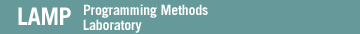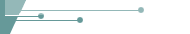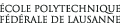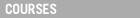Written Assignment
English only### Written Assignment

These exercises are to be handed in December 22nd, either during the course or by email to Burak. Most tasks here by courtesy of Fritz Henglein and Andrzej Filinski. For your convenience, there is a page with some figures from TAPL.

Arithmetic Expressions

Task 1) Recall the language of Arithmetic Expressions. Extend this language by two constructs "`plus t1 t2`" and "`equal t1 t2`". Give evaluation rules for the new constructs, so that they realize addition (of numerical values) and equality testing (on Nums and Bools), respectively. Evaluation should get stuck for ill-typed terms.

Task 2) Consider the extension with plus only. Show that evaluation always terminates, i.e., that any well-typed term reduces in a finite number of steps to a normal form.

Hint: consider a variant of the size function that weighs certain subterms heavier than others.

Lists and lambda calculus

Task 3) Consider the pure lambda-calculus, extended by numerical values `succ(..succ(zero))` and the `plus` construct of Task 1. A list `[t1,t2,t3]` can be coded by the lambda-term `\c.\n.c t1 (c t2(c t3 n))`. Without using general recursion (fix), define the following functions on such lambda-encoded lists.

 a) `sum`: adds the elements of a list b) `length`: determines the number of elements in a list c) `append`: concatenates two lists d) `map`: applies a function to every element of a list e) `reverse`: reverses a list

For each function give an example application and show how it evaluates to the desired result.

Typing Rules

Task 4) Consider the typing rule

 Γ |- `\x:T1. t x:T` Γ |- t:T
(T-Eta)

If this rule is added to pure simply typed lambda calculus (Fig 9-1, p.103, like Exercise 3 without let,fix,numbers, booleans, if) then does Lemma 9.3.1 (inversion of the typing relation) hold as stated? What about Theorem 9.3.3 (uniqueness of types)? Is the rule admissible, that is, whenever the premises can be derived, the conclusion can be derived also?

Subtyping

Task 5) Consider a type system with rules (T-Var), (T-Abs), (T-App), (S-Refl), (S-Trans), and (T-Sub) from Fig 15-1 (p. 186, TAPL), together with some unspecified collection of subtyping axioms at base types (e.g., Int <: Real). Show that, for typability in this system, (T-Eta) is equivalent to (S-Arrow). That is, show that a judgment Γ |- t : T is derivable in the system extended with (T-Eta) iff it is derivable in the system exteded with (S-Arrow).

(If you can't show the result in full generality, try for a weaker variant, such as only one direction of the biimplication, or a representative special case. Just be precise about what exactly you are showing.)

(Optional tasks give bonus points.)

Task 6) [optional] Write out the proof (in detail) of the progress theorem for FJ.

Task 7) [optional] Exercise 22.3.9 (pp. 325-326 of TAPL) (Write out constraint typing rules where a set of unused fresh variables F is threaded through a derivation). Prove that the system are equivalent, in an appropriate sense.